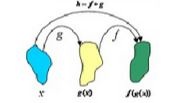Ontario Tech acknowledges the lands and people of the Mississaugas of Scugog Island First Nation.

We are thankful to be welcome on these lands in friendship. The lands we are situated on are covered by the Williams Treaties and are the traditional territory of the Mississaugas, a branch of the greater Anishinaabeg Nation, including Algonquin, Ojibway, Odawa and Pottawatomi. These lands remain home to many Indigenous nations and peoples.

We acknowledge this land out of respect for the Indigenous nations who have cared for Turtle Island, also called North America, from before the arrival of settler peoples until this day. Most importantly, we acknowledge that the history of these lands has been tainted by poor treatment and a lack of friendship with the First Nations who call them home.

This history is something we are all affected by because we are all treaty people in Canada. We all have a shared history to reflect on, and each of us is affected by this history in different ways. Our past defines our present, but if we move forward as friends and allies, then it does not have to define our future.

# Composition of Functions

The composition of two functions f and g is the new function h, where h(x) = f(g(x)), for all x in the domain of g such that g(x) is in the domain of f. The notation for function composition is h = f • g or h(x) = (f • g)(x) and is read as 'f of g of x'. The procedure is called composition because the new function is composed of the two given functions f and g, where one function is substituted into the other.## Finding the Composition

Although composition of functions is best illustrated with an example, let us summarize the key steps:

• rewrite f • g as f(g(x));
• replace g(x) with the function that it represents;
• evaluate f by replacing every x with the function that g(x) represents; and
• finally, if given a numerical value of x, evaluate the new function at this value by replacing all remaining x with the given value.

Note: Often f • g ≠ g • f and the two will have different domains. Also, be aware that you can take the composition  of more than two functions: e.g., f(g(k(x))).

Example: Given the function f(x) = x2 and g(x) = x + 3, find f(g(1)) and g(f(1)).

Solution:

f(g(1)) = f(x + 3)                               g(f(x)) = g(x2)

= (x +3)2                                                     = x2 + 3

f(g(1)) = (1 + 3)2                              g(f(1)) = 12 + 3

= 16                                                 = 4

Notice that  f • g ≠ g • f

Composition of Function Example 1:

Composition of Function Example 2:

Composition of Function Example 3:

Composition of Function Example 4:

Composition of Function Example 5:

Composition of Function Example 6: